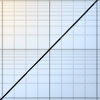Search by Topic

Resources tagged with Exponential function similar to Exponential Trend:

Filter by: Content type:
Age range:
Challenge level:

There are 5 results

Broad Topics > Functions and Graphs > Exponential functionExponential Trend

Age 16 to 18 Challenge Level:

Find all the turning points of y=x^{1/x} for x>0 and decide whether each is a maximum or minimum. Give a sketch of the graph.Hyperbolic Thinking

Age 16 to 18 Challenge Level:

Explore the properties of these two fascinating functions using trigonometry as a guide.Gosh Cosh

Age 16 to 18 Challenge Level:

Explore the hyperbolic functions sinh and cosh using what you know about the exponential function.Integral Equation

Age 16 to 18 Challenge Level:

Solve this integral equation.What Are Complex Numbers?

Age 16 to 18

This article introduces complex numbers, brings together into one bigger 'picture' some closely related elementary ideas like vectors and the exponential and trigonometric functions and. . . .